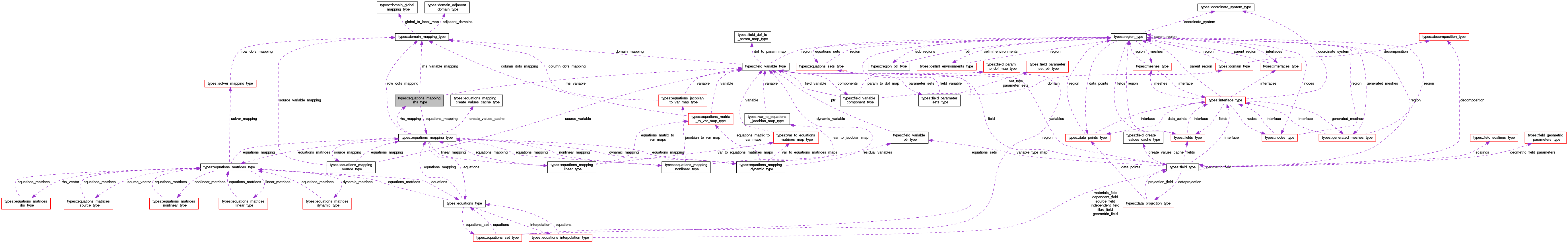OpenCMISS-Iron Internal API Documentation
types::equations_mapping_rhs_type Type Reference

Contains information on the equations mapping for a RHS i.e., how a field variable is mapped to the RHS vector for the equations set of this equations mapping. More...

Collaboration diagram for types::equations_mapping_rhs_type:[legend]

## Public Attributes

type(equations_mapping_type), pointer equations_mapping
A pointer to the equations mapping. More...

integer(intg) rhs_variable_type
The variable type number mapped to the RHS vector. More...

type(field_variable_type), pointer rhs_variable
A pointer to the variable that is mapped to the RHS vector. More...

type(domain_mapping_type), pointer rhs_variable_mapping
A pointer to the RHS variable domain mapping. More...

real(dp) rhs_coefficient
The multiplicative coefficient applied to the RHS vector. More...

integer(intg), dimension(:), allocatable rhs_dof_to_equations_row_map
RHS_DOF_TO_EQUATIONS_ROW_MAP(residual_dof_idx). The mapping from the rhs_dof_idx'th RHS dof in the rhs variable to the equations row. More...

integer(intg), dimension(:), allocatable equations_row_to_rhs_dof_map
EQUATIONS_ROW_TO_RHS_DOF_MAP(row_idx). The mapping from the row_idx'th row of the equations to the RHS dof. More...

## Detailed Description

Contains information on the equations mapping for a RHS i.e., how a field variable is mapped to the RHS vector for the equations set of this equations mapping.

Definition at line 1635 of file types.f90.

## Member Data Documentation

 type(equations_mapping_type), pointer types::equations_mapping_rhs_type::equations_mapping

A pointer to the equations mapping.

Definition at line 1636 of file types.f90.

 integer(intg), dimension(:), allocatable types::equations_mapping_rhs_type::equations_row_to_rhs_dof_map

EQUATIONS_ROW_TO_RHS_DOF_MAP(row_idx). The mapping from the row_idx'th row of the equations to the RHS dof.

Definition at line 1642 of file types.f90.

 real(dp) types::equations_mapping_rhs_type::rhs_coefficient

The multiplicative coefficient applied to the RHS vector.

Definition at line 1640 of file types.f90.

 integer(intg), dimension(:), allocatable types::equations_mapping_rhs_type::rhs_dof_to_equations_row_map

RHS_DOF_TO_EQUATIONS_ROW_MAP(residual_dof_idx). The mapping from the rhs_dof_idx'th RHS dof in the rhs variable to the equations row.

Definition at line 1641 of file types.f90.

 type(field_variable_type), pointer types::equations_mapping_rhs_type::rhs_variable

A pointer to the variable that is mapped to the RHS vector.

Definition at line 1638 of file types.f90.

 type(domain_mapping_type), pointer types::equations_mapping_rhs_type::rhs_variable_mapping

A pointer to the RHS variable domain mapping.

Definition at line 1639 of file types.f90.

 integer(intg) types::equations_mapping_rhs_type::rhs_variable_type

The variable type number mapped to the RHS vector.

Definition at line 1637 of file types.f90.